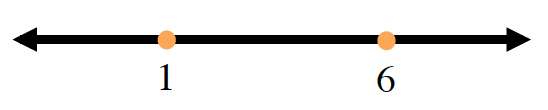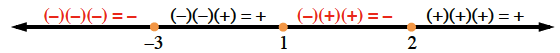### Home > PC3 > Chapter 4 > Lesson 4.3.1 > Problem4-109

4-109.

Solve the inequalities below and express the solutions in both interval and inequality notation.

1. $x^2-7x\le-6$

Solve this as an equation. Being by setting one side equal to $0$.

$x^2−7x+6=0$

Factor and determine the "boundary points".

$(x−6)(x−1)=0$Test a point in each interval to see whether or not it makes the original inequality true.

1. $(x-2)(x-1)(x+3)<0$